## Numerical Exampe of K Nearest Neighbor Algorithm

Here is step by step on how to compute K-nearest neighbors KNN algorithm:

1. Determine parameter K = number of nearest neighbors
2. Calculate the distance between the query-instance and all the training samples
3. Sort the distance and determine nearest neighbors based on the K-th minimum distance
4. Gather the category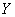of the nearest neighbors
5. Use simple majority of the category of nearest neighbors as the prediction value of the query instance

We will use again the previous example to calculate KNN by hand computation. If you want to download the MS excel companion of this tutorial, click here

Example

We have data from the questionnaires survey (to ask people opinion) and objective testing with two attributes (acid durability and strength) to classify whether a special paper tissue is good or not. Here is four training samples

 X1 = Acid Durability (seconds) X2 = Strength (kg/square meter) Y = Classification 7 7 Bad 7 4 Bad 3 4 Good 1 4 Good

Now the factory produces a new paper tissue that pass laboratory test with X1 = 3 and X2 = 7. Without another expensive survey, can we guess what the classification of this new tissue is?

1. Determine parameter K = number of nearest neighbors

Suppose use K = 3

2. Calculate the distance between the query-instance and all the training samples

Coordinate of query instance is (3, 7), instead of calculating the distance we compute square distance which is faster to calculate (without square root)

 X1 = Acid Durability (seconds) X2 = Strength (kg/square meter) Square Distance to query instance (3, 7) 7 7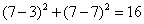7 4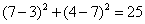3 4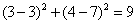1 4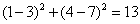3. Sort the distance and determine nearest neighbors based on the K-th minimum distance

 X1 = Acid Durability (seconds) X2 = Strength (kg/square meter) Square Distance to query instance (3, 7) Rank minimum distance Is it included in 3-Nearest neighbors? 7 73 Yes 7 44 No 3 41 Yes 1 42 Yes

4. Gather the categoryof the nearest neighbors. Notice in the second row last column that the category of nearest neighbor (Y) is not included because the rank of this data is more than 3 (=K).

 X1 = Acid Durability (seconds) X2 = Strength (kg/square meter) Square Distance to query instance (3, 7) Rank minimum distance Is it included in 3-Nearest neighbors? Y = Category of nearest Neighbor 7 73 Yes Bad 7 44 No - 3 41 Yes Good 1 42 Yes Good

5. Use simple majority of the category of nearest neighbors as the prediction value of the query instance

We have 2 good and 1 bad, since 2>1 then we conclude that a new paper tissue that pass laboratory test with X1 = 3 and X2 = 7 is included in Good category.

Preferable reference for this tutorial is

Teknomo, Kardi. K-Nearest Neighbors Tutorial. https:\\people.revoledu.com\kardi\tutorial\KNN\